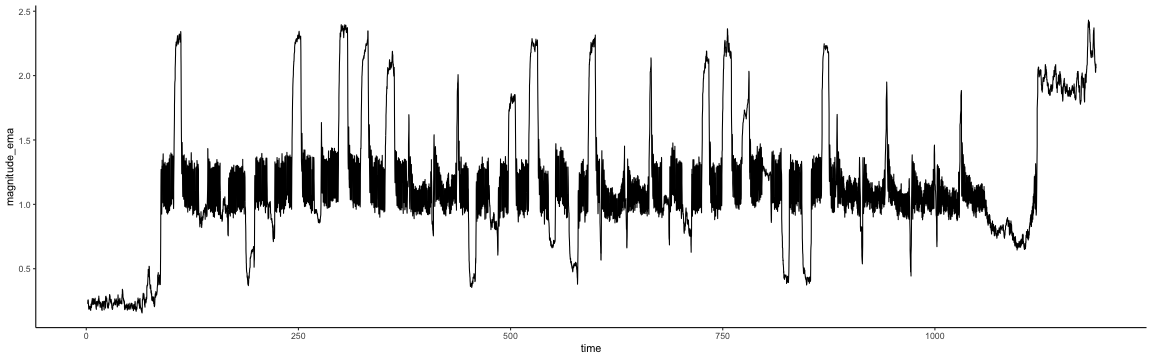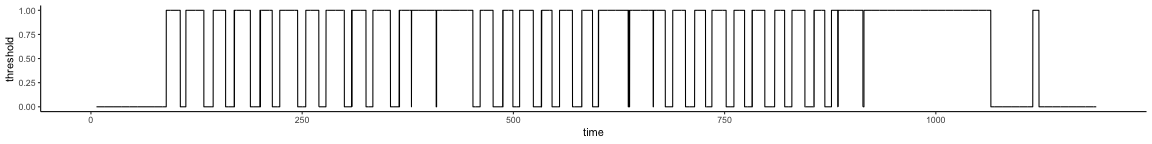Martin Isaksson

Using a PocketLab put on the top of a washing machine, we measure the magnetic field generated by the motor. The magnitude of this vector is used to detect if the motor is on, and when the motor has been off for some time, we say that the cycle is finished.

## Checkpoint

#library(checkpoint)
#checkpoint("2015-08-26")


## Libraries

library(dplyr)
library(tidyr)
library(ggplot2)
library(TTR)
library(printr)

#library(mime)


Read data from PocketLab CSV and calculate magnitude from x, y and z components. Then use an Exponential Moving Average filter to remove the trend (seems like the sensor does not return to zero when the washing machine motor turns off).

wash <- read.csv("data/Magnetic field368141090.csv", col.names=c("time", "x", "y","z")) %>%
mutate(
magnitude = sqrt(x^2 + y^2 + z^2), # Calculate magnitude
magnitude_ema = EMA(magnitude,20), # Exponential Moving Average
magnitude_adj = magnitude - magnitude_ema, # Remove trend
magnitude_abs = magnitude_adj %>% abs %>% EMA(., 50),
magnitude_abs = scale(magnitude_abs) %>% as.vector, # Absolute value and more EMA
threshold = ifelse(magnitude_abs > 0, 1, 0)
) %>%
filter(time < 1190)


0.000.06103520.0610352-0.30517580.3171480NANANANA
0.020.0610352-0.0305176-0.12207030.1398491NANANANA
0.080.15258790.0915527-0.33569340.3799408NANANANA
0.160.03051760.12207030.06103520.1398491NANANANA
0.260.00000000.0305176-0.03051760.0431584NANANANA
0.360.00000000.0000000-0.39672850.3967285NANANANA

Rearrange and plot x,y and z components.

wash2 <- wash %>% select(-matches("magnitude"), -threshold) %>% gather("axis", "value",-time)

ggplot(wash2, aes(x=time, y=value, color=axis)) +
geom_line() +
theme_classic() +
facet_grid(axis~.) +
scale_colour_brewer(palette = "Set1")Plot the magnitude

ggplot(wash, aes(x=time, y=magnitude)) +
geom_line() +
theme_classic()Plot the magnitude trend

ggplot(wash, aes(x=time, y=magnitude_ema)) +
geom_line() +
theme_classic()

## Warning: Removed 19 rows containing missing values (geom_path).Plot the adjusted magnitude (removed magnitude trend).

ggplot(wash, aes(x=time, y=magnitude_adj)) +
geom_line() +
theme_classic()

## Warning: Removed 19 rows containing missing values (geom_path).Plot the normalized absolute magnitude.

ggplot(wash, aes(x=time, y=magnitude_abs)) +
geom_line() +
theme_classic()

## Warning: Removed 68 rows containing missing values (geom_path).Using zero as a threshold, plot on or off status.

ggplot(wash, aes(x=time, y=threshold)) + geom_line()+ theme_classic()

## Warning: Removed 68 rows containing missing values (geom_path).## Suggested citation

If you would like to cite this work, here is a suggested citation in BibTeX format.